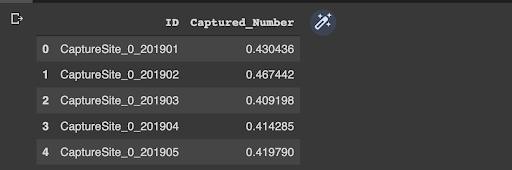Turtle Rescue Forecast Challenge Tutorial
Technical · 26 May 2022, 08:02

Welcome to our tutorial on one of Zindi’s most popular knowledge challenges - the Sea Turtle Rescue Forecast Challenge. This article will take you through the basic procedure on how best to approach this task, show you some basic techniques you can use and finally show you how to create and upload your submission to Zindi for scoring.

You can use this Colab notebook to follow along with this tutorial and make your first submission.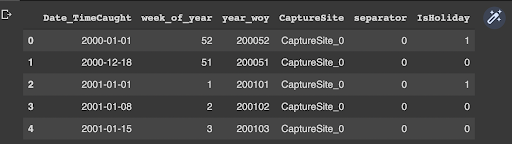### The task we are trying to solve

The objective of this challenge is to forecast the number of turtles rescued in a week per beach in Kenya.

Forecasting is the art of making predictions based on time-series data. A time series is a data set that tracks a sequence of observations over time. The only difference between prediction and forecasting is that we consider the temporal dimension.

Prediction is about fitting a shape that gets as close to the data as possible. In forecasting, the future data is entirely unavailable, so you are required to extrapolate from what has already happened.

Each time a fisherman in Kenya catches a turtle by mistake, they take the turtle to Local Ocean Conservation to be assessed; the fisherman also receives a small remuneration.

The business objective of this challenge is to help the LOC to plan their staff schedules and budget by better understanding the volume of turtles they can expect to be delivered in a given week.

### Analysis question

Can we forecast the number of turtles rescued per site per week?

### Data understanding

Data understanding is a step that gives a blueprint for what to work on in data preparation. It provides us with an idea of which variables we should focus on and transform (if need be) to improve the predictive power of our machine learning or deep learning models.

Here, we need to check the data’s quality and completeness, explore variables and their relationship to one another and the problem we are trying to solve.

We need to access the different variables in the dataset and hypothesise how likely they are to affect the number of turtles rescued per week.

We are provided with 4 different data files to work with:

1. variable_definitions.csv: This file describes the different variables in the data.
2. sampleSubmission.csv: This file shows the structure of your submission file.
3. train.csv: This file contains relevant data/variables that will help train the final model; It can be split into train and test to test locally.
4. CaptureSite_category.csv: This file contains the capture sites and their category.

Hint: Since this is a time forecasting challenge, we’ll likely prioritise the time variables.

To get a clearer picture, it’s important to create a structural hypothesis with general knowledge to identify which variables are likely to affect the final output.

In this challenge, we’ll focus on train.csv and CaptureSite_category.csv since they contain the relevant data sets that we need.

### Exploratory data analysis (EDA)

Missing values

Code to check for missing values.

`# Check for missing values and duplicates`
```train.isnull().sum().any(), capture_site_category.isnull().sum().any()

```

The training dataset has some missing values while the captur_site_category doesn't have any missing values.

Let us investigate further and check exactly which columns have missing values.

`# Plot showing missing values in train set`
`ax = train.isna().sum().sort_values().plot(kind = 'barh', figsize = (9, 10))`
`plt.title('Percentage of Missing Values Per Column in Train Set', fontdict={'size':15})`
`for p in ax.patches:`
`percentage ='{:,.0f}%'.format((p.get_width()/train.shape)*100)`
`width, height =p.get_width(),p.get_height()`
`x=p.get_x()+width+0.02`
`y=p.get_y()+height/2`
`ax.annotate(percentage,(x,y))`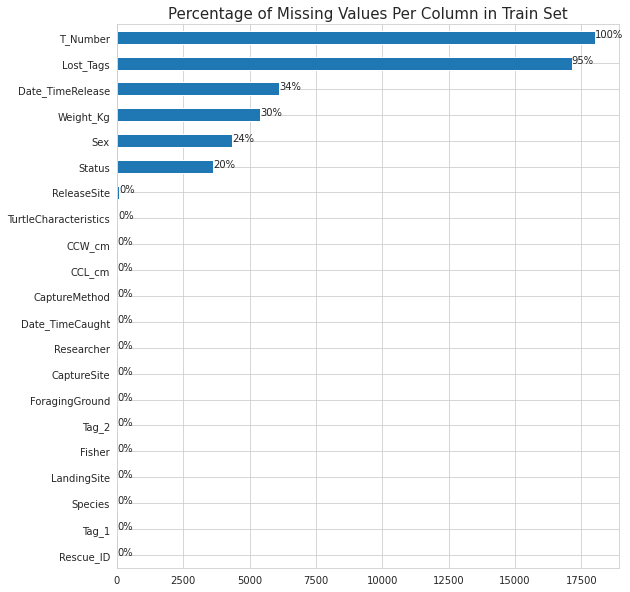The T_Number column has 100% missing values and the Lost_Tags column has 95% missing values. This columns have to be dropped as they dont have any meaningful information

Let us check how many rescue sites there are:

`# View the list of unique Capture Sites sites`
`display(capture_site_category.CaptureSite.unique())`
`# Number of unique sites`
`print(f'\n Number of unique sites: {capture_site_category.CaptureSite.nunique()}')`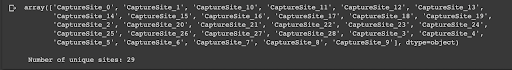We can theorise what this means. There are probably 29 areas where turtles are normally caught, maybe 29 different beaches. These 'beaches' can be categorised into 5 possible areas, maybe rocky areas, north beach, south beach... This can be summarised further into 2 Types, maybe breeding site or not breeding site.

This is only a theory but something we can work with.

We can compare CaptureSiteCategory with Train and merge these files. To do this we need to do some editing to the train file before we merge.

`# Data wrangling to create training and testing datasets`
`sample_submission["year_woy"]=(sample_submission.ID.apply(lambda x: x.split("_")[-1])).astype(int)`
`sample_submission["CaptureSite"]=sample_submission.ID.apply(lambda x: ("_").join(x.split("_")[0:-1]))`
`sample_submission.head()`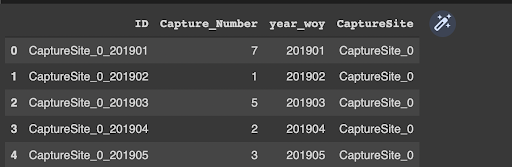Then we concatenate week of year and capture site in the training dataset to the submission dataset.

We also group the data by capture site and taking the minimum week of year.

`keys=pd.concat([train[["year_woy","CaptureSite"]],sample_submission[["year_woy","CaptureSite"]]])`
`CaptureSite_min_year_woy=keys.groupby("CaptureSite").year_woy.min().rename("year_woy").reset_index()`

We then join the cleaned datasets together

`# Joining the cleaned datasets together`
`final_data=[]`
`for site , year_woy in zip(CaptureSite_min_year_woy.CaptureSite.values,CaptureSite_min_year_woy.year_woy.values) :`
`one_site_df=range_year_woy[range_year_woy.year_woy>=year_woy]`
`one_site_df["CaptureSite"]=site`
`final_data.append(one_site_df)`
`final_data=pd.concat(final_data, ignore_index=True)`
```final_data.head()

The next step is extracting the target variable from the engineered dataset and also filling in the missing values with 0.

# Extracting the target variable from the dataset
Target=train.groupby(["year_woy","CaptureSite"]).CaptureSite.count().rename("Capture_Number").reset_index()
final_data=final_data.merge(Target,on=["year_woy","CaptureSite"],how="left")

# Fill in missing values
final_data.Capture_Number.fillna(0,inplace=True)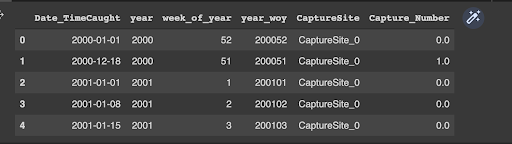Next step: Separate the training set from the test set.

```# Separating the training set and testing set
train=final_data[final_data.year<2019].reset_index(drop = True)
test=final_data[final_data.year==2019].reset_index(drop = True)

To engineer new features efficiently, we concatenate the training and test datasets together.```

We then use fast.ai library to add date features together with their cyclic date features.

```We can also engineer new features by taking into account days which are holidays in Kenya. You might get that more turtles are captured during holidays when compared to normal days.

#  Holiday
from pandas.tseries.holiday import *

class KenyaHoliday(AbstractHolidayCalendar):
rules = [
Holiday('New Year', month=1, day=1, observance=sunday_to_monday),
Holiday('Good Friday', month=1, day=1, offset=[Easter(), Day(-2)]),
Holiday('Easter Monday', month=1, day=1, offset=[Easter(), Day(1)]),
Holiday('Workers Day', month=5, day=1,observance=sunday_to_monday),
Holiday('Huduuma', month=10, day=10, observance=sunday_to_monday),
Holiday('Masuja', month=10, day=20, observance=sunday_to_monday),
Holiday('Jumaji Day', month=12, day=12, observance=sunday_to_monday),
Holiday('Goodwill Day', month=12, day=26, observance=sunday_to_monday),
Holiday('Christmas', month=12, day=25)
]

hol= KenyaHoliday()
myholidays =hol.holidays(start=train_test.Date_TimeCaught .min(), end = train_test.Date_TimeCaught.max())
hol_day = pd.Series(myholidays)

## Create instance of holiday in a new column
train_test['IsHoliday'] = np.where(train_test.Date_TimeCaught.isin(hol_day) ,1,0)
```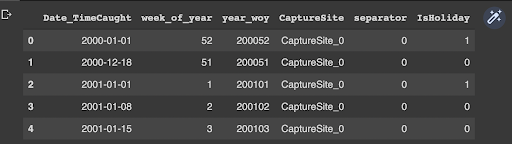### Modelling

In this challenge, we will use the CatBoost algorithm which is based on gradient boosting decision trees. Gradient boosting is a Machine learning technique used in classification and regression tasks.

Boosting is a method of converting weak learners into strong learners - what happens is the technique combines models in order to produce one optimal predictive model.

We use a five kfold validation technique to train our catboost regressor model.

```# Select X and y features
X = train.drop(['id', 'target'], axis = 1)
y = train.target
tess = test.drop('id', axis = 1)

# KFold Validation
folds = KFold(n_splits = 5, shuffle=True, random_state = 42)
```

After training, we get an average RMSE of 1.247.

The chart below shows the most important features that contributed to the RMSE above.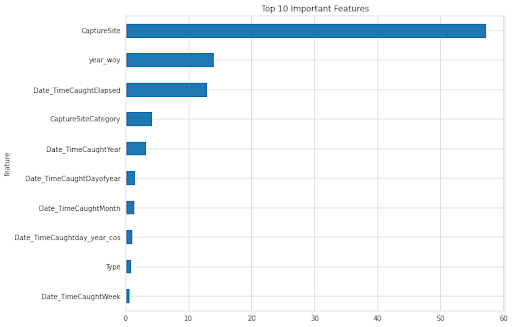Finally we can create the submission file and upload it to Zindi for scoring.

```# Creating a submission file
# Average predictions
mean_preds = np.mean(predictions, axis = 1)
sub_df = pd.DataFrame({'ID': test.id, 'Captured_Number': preds})